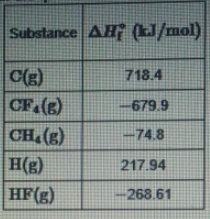# Problem: Part ACalculate the enthalpy change, ΔH, for the expansion of methaneCH4(g) → C(g) + 4H(g)Part BCalculate the enthalpy change, ΔH for the reverse of the formation of methane.CH4(g) → C(s) + 2 H2(g)Part CSuppose that 0.570 mol of methane CH4(g) is reacted with 0.720 mol of fluorine, F2(g), forming CF4(g) and HF (g) as sole products. Assuming that the reaction occurs at constant pressure, how much heat is released?

###### FREE Expert Solution

Part A) Calculate the enthalpy change, ΔH, for the expansion of methane:

We can use the following equation to solve for ΔH˚rxn:

Note that we need to multiply each ΔH˚f by the stoichiometric coefficient since ΔH˚f is in kJ/mol.

Also, note that ΔH˚f for elements in their standard state is 0.

CH4(g) → C(g) + 4 H(g)

83% (77 ratings)###### Problem Details

Part A

Calculate the enthalpy change, ΔH, for the expansion of methane

CH4(g) → C(g) + 4H(g)

Part B

Calculate the enthalpy change, ΔH for the reverse of the formation of methane.

CH4(g) → C(s) + 2 H2(g)

Part C

Suppose that 0.570 mol of methane CH4(g) is reacted with 0.720 mol of fluorine, F2(g), forming CF4(g) and HF (g) as sole products. Assuming that the reaction occurs at constant pressure, how much heat is released?Frequently Asked Questions

What scientific concept do you need to know in order to solve this problem?

Our tutors have indicated that to solve this problem you will need to apply the Enthalpy of Formation concept. You can view video lessons to learn Enthalpy of Formation. Or if you need more Enthalpy of Formation practice, you can also practice Enthalpy of Formation practice problems.